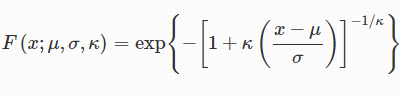# How can i calculate non-stationary precipitation index(NSPI)?

How can i calculate non-stationary precipitation index(NSPI) in Excel? How can i set GAMLSS model easily?

In the procedure of calculation of the Standard Precipitation Index, the Gamma distribution usually has been used. This comes from McKee et al. (1993), but some researchers calculate it by other distributions that can be applied to the index calculation. Many distribution functions are compared for SPI and SPEI. To calculate the non-stationary precipitation index(NSPI), the GEV distribution is used. GEV distribution is expressed as below Eq:The parameter equations proposed by Coles (2001) and Katz et al. (2002) are expressed by Eq.

We don't have any tool that calculates the non-stationary precipitation index(NSPI) but all of the users of DMAP V2.0 can order to add the new index in this tool. So you can buy this tool and then order to add the non-stationary precipitation index(NSPI) to the tool.